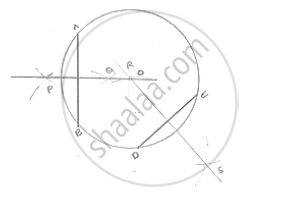# Draw a Circle with Centre at Point O. Draw Its Two Chords Ab and Cd Such that Ab is Not Parallel to Cd. Draw the Perpendicular Bisectors of Ab and Cd. at What Point Do They Intersect? - Mathematics

Draw a circle with centre at point O. Draw its two chords AB and CD such that AB is not
parallel to CD. Draw the perpendicular bisectors of AB and CD. At what point do they
intersect?

#### SolutionSteps of construction:
1. With center O and any radius, draw a circle
2. Draw two chords AB and CD.
3. With center A and radius more than 1/2AB ,draw arcs, one on each side of AB
4. With center B and same radius draw arcs cutting previous arcs at P and Q respectively.
5. Join PQ
6. With center D and radius more than 1/2 DC draw arcs, one on each side of DC
7. With center C and same radius, draw arcs cutting previous arcs at R and S respectively
8. Join RS
Both perpendicular bisector PQ and RS intersect each other at the center O of he circle.

Concept: Basic Constructions
Is there an error in this question or solution?

#### APPEARS IN

RD Sharma Mathematics for Class 9
Chapter 16 Constructions
Exercise 16.1 | Q 4 | Page 4

Share